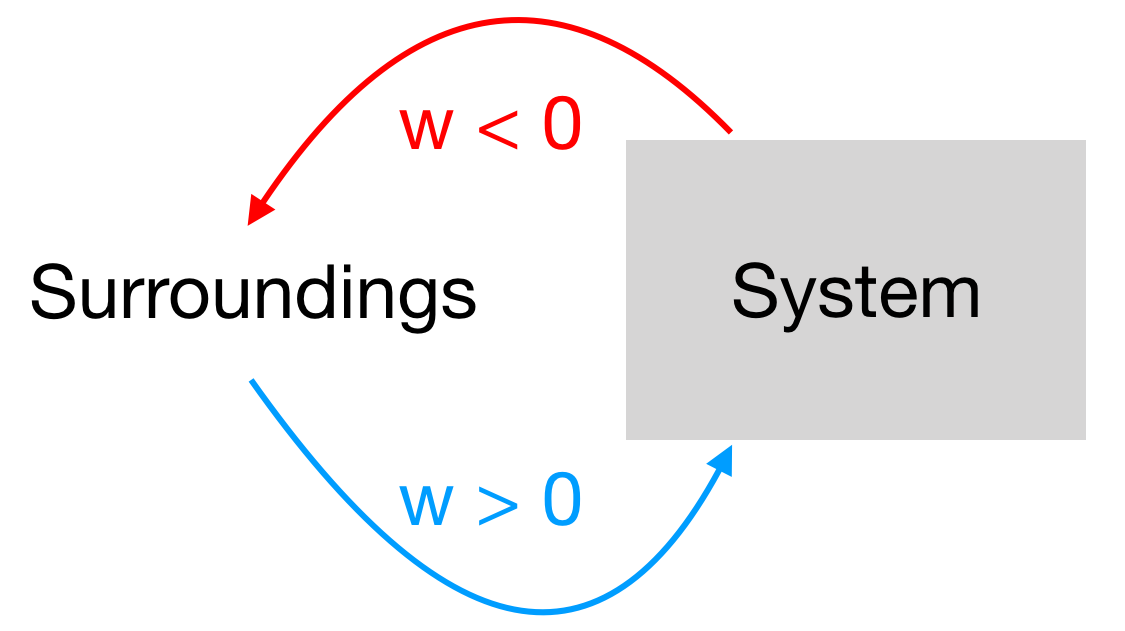# Problem: At constant pressure, which of these systems do work on the surroundings? A. 2A(g) + 3B(g) → 4C(g)B. A(s) + B(s) → C(g)C. 2A(g) + 2B(g) → 3C(g)D. 2A(g) +B(g) → 4C(g)E. More than one of the above

🤓 Based on our data, we think this question is relevant for Professor Jurisch's class at UIC.

###### FREE Expert Solution

Recall: When the system does work on the surroundings, the sign for work (w) is negative (w <  0). On the other hand, work is positive (w > 0) if the surroundings do work on the system. To illustrate###### Problem Details

At constant pressure, which of these systems do work on the surroundings?

A. 2A(g) + 3B(g) → 4C(g)

B. A(s) + B(s) → C(g)

C. 2A(g) + 2B(g) → 3C(g)

D. 2A(g) +B(g) → 4C(g)

E. More than one of the above

What scientific concept do you need to know in order to solve this problem?

Our tutors have indicated that to solve this problem you will need to apply the Internal Energy concept. You can view video lessons to learn Internal Energy. Or if you need more Internal Energy practice, you can also practice Internal Energy practice problems.

What is the difficulty of this problem?

Our tutors rated the difficulty ofAt constant pressure, which of these systems do work on the ...as medium difficulty.

How long does this problem take to solve?

Our expert Chemistry tutor, Sabrina took 5 minutes undefined to solve this problem. You can follow their steps in the video explanation above.

What professor is this problem relevant for?

Based on our data, we think this problem is relevant for Professor Jurisch's class at UIC.